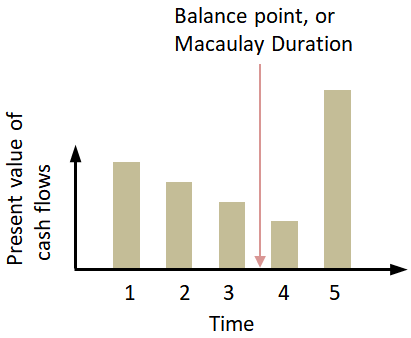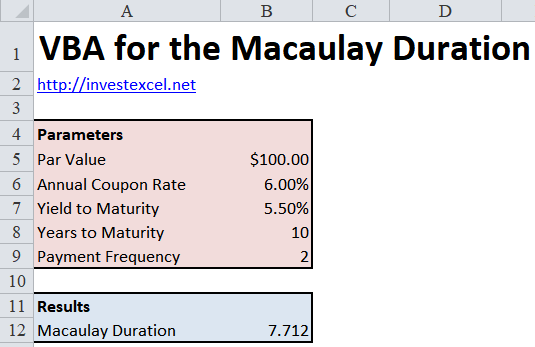# VBA for the Macaulay Duration

The Macaulay Duration is a fundamental characteristic of a bond, and is the weighted average of the time until each payment is received.

The VBA can be found further down. But first let’s talk about what the Macaulay Duration actually means.

An appropriate analogy is a scale, with the present value of each coupon payment placed on the scale.On the right of the scale is the payment of the principal of the bond. The balance point (or duration) is the time-weighted average number of years until the present value of the bond’s cash flows equals the amount paid for the bond.

## Formula

The Macaulay Duration defined by this equation.

• P is the bond price
• C is the coupon
• n is the number of periods
• M is the face value

The formula looks complicated, but is easy to understand. You just

• divide the present value of each periodic cash flow by the present value of the bond (bear in mind that in the final year, the principal is also paid in additional to the coupon)
• multiply each by the year in which the payment is made
• and add the total together

## What Affects a Bond’s Duration

What does the equation above tell us about how the duration changes with respect to its parameters?

• As the time to maturity (i.e. the number of payments n) increases, the duration increases. This pulls the balance point on the scale to the right
• As the coupon increases, the duration decreases, pulling the balance point to the left
• As the yield to maturity i increases, the balance point is pulled to the left and the duration decreases

Here’s a VBA function that calculates the Macaulay Duration.

```Function MacDur( _ ByVal ParValue As Double, _ ByVal TimeToMaturity As Double, _ ByVal CouponRate As Double, _ ByVal YieldtoMaturity As Double, _ ByVal Frequency As Double) As Double   Dim i As Integer, PresentValue As Double   PresentValue = CouponRate * ParValue / Frequency * (1 - 1 / (1 + YieldtoMaturity / Frequency) ^ (TimeToMaturity * Frequency)) / (YieldtoMaturity / Frequency) + ParValue / (1 + YieldtoMaturity / Frequency) ^ (TimeToMaturity * Frequency)   MacDur = 0   For i = 1 To Frequency * TimeToMaturity MacDur = MacDur + i / Frequency * ParValue * CouponRate / Frequency / (1 + YieldtoMaturity / Frequency) ^ i Next   MacDur = (MacDur + TimeToMaturity * ParValue / (1 + YieldtoMaturity / Frequency) ^ (Frequency * TimeToMaturity)) / PresentValue   End Function```

The VBA function lets you alter the could payment frequency (the majority of bonds pay a coupon two times a year). The code gives the same result as Excel’s built-in MDURATION function.Here’s a spreadsheet that implements the VBA. The VBA isn’t locked away – feel free to use and abuse the code in any way you want.

Spreadsheet with VBA for Macaulay Duration